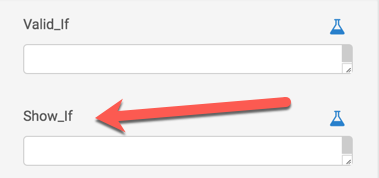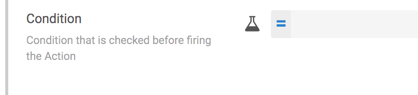# Yes/No expressions

Write expressions where the result is either TRUE or FALSE

Yes/No expressions produce a result that's either `TRUE` (Yes) or `FALSE` (No). These expressions are generally used in AppSheet where you need an `IF` statement. For example, when you see a Show_IF or Condition field.

The following shows a Show_If condition:The following shows a general IF Condition field:## Yes/No operators

Yes/No expressions use operators that return a `TRUE` or `FALSE` result displayed as a `Yes` or `No` in AppSheet. Yes/No operators include:

### Comparison operators

AppSheet supports comparison conditions by using comparison operators with two expression parameters that have comparable types. These rely on comparing two or more parameters. The expression will either have a `TRUE` or `FALSE` result, depending on whether the comparison is valid. For example, `5 > 2` is valid, but `5 > "Hello"` is not valid.

• Equals: `=`
• Not Equals: `<>`
• Greater Than: `>`
• Greater Than or Equals: `>=`
• Less Than: `<`
• Less Than or Equals: `<=`

### Composition operators

This is a powerful way to combine multiple conditions. If you wrap multiple conditions with `AND`, `OR`, `NOT`, you can compare a greater number of things. These rely on the conditions of multiple components and can be used to determine what to do when something is true or false.

#### AND({condition 1},{condition 2},{condition 3},..,{condition n})

If all the conditions in the `AND` expression are true, then the result of the expression will be `TRUE` (Yes), if any of the conditions are not true, the result will be `FALSE` (No). Separate each condition with a comma. Each condition is an expression in itself, so be aware of how the expressions are nested.

#### OR({condition 1},{condition 2},{condition 3},..,{condition n}) (OR)

If any of the conditions in the expression is true then the `OR` expression will result in `TRUE`, otherwise it will be `FALSE`. Each condition is separated by a comma.

#### NOT({condition})  (NOT)

If the condition result is true, then wrapping it in `NOT()` results in `FALSE`. If the condition is `FALSE`, then wrapping it in `NOT()` results in `TRUE`.

## Other operators

AppSheet supports the following additional operators:

• `ISBLANK({*})` returns `TRUE` if an expression is empty
• `CONTAINS({text_1},{text_2})` returns `TRUE` if `text_1` contains `text_2`
• `IN({*},{List})` returns true if a value is in a list

## Common and complex expressions

These are some examples of commonly used Yes/No expressions in AppSheet.

### Common expressions

If you wanted to do something when the value of column `Color` is green and it was completed today, here's the expression you would use:

`AND([Color]="Green",[CompleteDate]>TODAY())`

If you wanted to see whether there are any oranges recorded in the column `Fruit`, you would use this expression:

`CONTAINS([Fruit],"Oranges")`

### Complex expressions

These are some examples of more complex operations where more parameters are included.

`OR(([Price]*[Quantity])>\$10,000.00,[Price]>\$100.00)`

`OR(CONTAINS([Fruit],"Oranges"),CONTAINS([Fruit],"Apples"),CONTAINS([Fruit],"Bananas"))`

You can use column names, constants (like dates, names, or other values), and other mathematical operations to build your expression. The only condition is that the result is either `TRUE` or `FALSE` (Yes/No).

Expressions may be used in various AppSheet features - App formulas and initial values, Virtual columns and Column constraints (`Valid_If`, `Show_If`, `Required_If`) - to customize app behavior and provide advanced functionality.

Expressions in this article align with the Yes/No Expressions section of the Expression Assistant in the Editor.